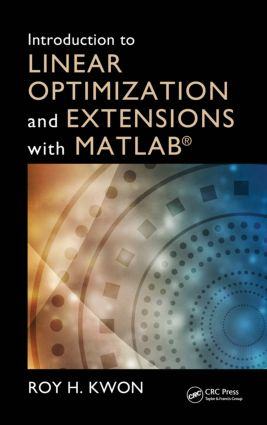Introduction to Linear Optimization and Extensions with MATLAB®

1st Edition

CRC Press

362 pages | 37 B/W Illus.

Hardback: 9781439862636
pub: 2013-09-05
\$130.00
x
eBook (VitalSource) : 9780429169458
pub: 2013-09-05
from \$65.00

FREE Standard Shipping!

Description

Filling the need for an introductory book on linear programming that discusses the important ways to mitigate parameter uncertainty, Introduction to Linear Optimization and Extensions with MATLAB® provides a concrete and intuitive yet rigorous introduction to modern linear optimization. In addition to fundamental topics, the book discusses current linear optimization technologies such as predictor-path following interior point methods for both linear and quadratic optimization as well as the inclusion of linear optimization of uncertainty i.e. stochastic programming with recourse and robust optimization.

The author introduces both stochastic programming and robust optimization as frameworks to deal with parameter uncertainty. The author’s unusual approach—developing these topics in an introductory book—highlights their importance. Since most applications require decisions to be made in the face of uncertainty, the early introduction of these topics facilitates decision making in real world environments. The author also includes applications and case studies from finance and supply chain management that involve the use of MATLAB.

Even though there are several LP texts in the marketplace, most do not cover data uncertainty using stochastic programming and robust optimization techniques. Most emphasize the use of MS Excel, while this book uses MATLAB which is the primary tool of many engineers, including financial engineers. The book focuses on state-of-the-art methods for dealing with parameter uncertainty in linear programming, rigorously developing theory and methods. But more importantly, the author’s meticulous attention to developing intuition before presenting theory makes the material come alive.

Reviews

"The book goes beyond a `cookbook' for linear optimization in Matlab; instead it outlines and explains the theory behind each linear optimization technique and a number of essential theorems are provided and proven. This greatly helps the reader understand why each technique works and how it is implemented in the Matlab software. Computational projects suggested in the book can also assist students with the practical implementation of the techniques in real-life applications.

—Efstratios Rappos (Aubonne) in Zentralblatt, MATH 1287

Linear Programming

Introduction

General Linear Programming Problems

More Linear Programming Examples

Exercises

Computational Project

Geometry of Linear Programming

Introduction

Geometry of the Feasible Set

Extreme Points and Basic Feasible Solutions

Resolution (Representation) Theorem

Exercises

The Simplex Method

Introduction

Simplex Method Development

Generating an Initial Basic Feasible Solution (Two-Phase and Big M Methods)

Degeneracy and Cycling

Revised Simplex Method

Complexity of the Simplex Method

Simplex Method MATLAB Code

Exercises

Duality Theory

Introduction

Motivation for Duality

Forming the Dual Problem for General Linear Programs

Weak and Strong Duality Theory

Complementary Slackness

Duality and the Simplex Method

Economic Interpretation of the Dual

Sensitivity Analysis

Exercises

Dantzig-Wolfe Decomposition

Introduction

Decomposition for Block Angular Linear Programs

Master Problem Reformulation

Restricted Master Problem and the Revised Simplex Method

Dantzig-Wolfe Decomposition

Dantzig-Wolfe MATLAB Code

Exercises

Interior Point Methods

Introduction

Linear Programming Optimality Conditions

Primal-Dual Interior Point Strategy

The Predictor-Corrector Variant of the Primal-Dual Interior Point Method

Primal-Dual Interior Point Method in MATLAB

Exercises

Introduction

QP Model Structure

QP Application: Financial Optimization

Exercises

Linear Optimization under Uncertainty

Introduction

Stochastic Programming

More Stochastic Programming Examples

Robust Optimization

Exercises

A Linear Algebra Review

Bibliography

Roy H Kwon is a professor at University of Toronto - St. George Campus, Canada.